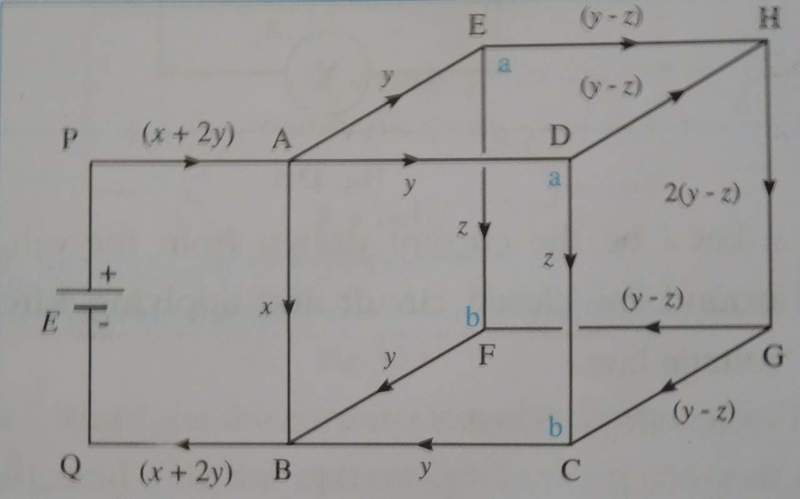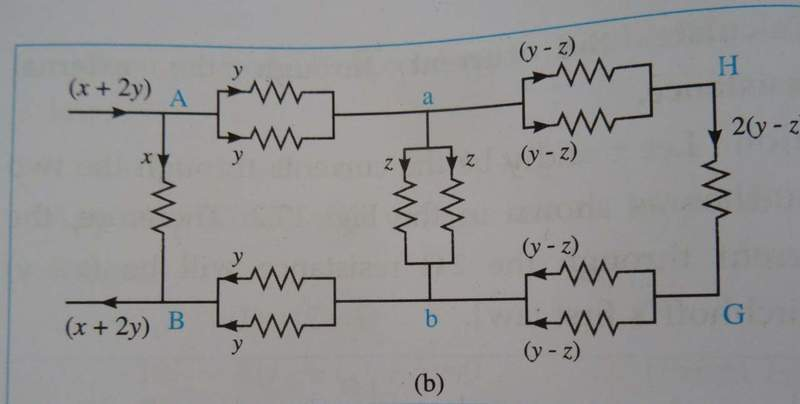# Cube of resistors

## Homework Statement

Here is a cube of resistors with each resistance of value "R".Find the equivalent resistance between A and B.## The Attempt at a Solution

My book says the equivalent circuit of the cube is :But i don't think it is correct.They have made parallel combinations which don't seem right.Is the book correct??

#### Attachments

phinds
Gold Member
you need to make your figures smaller. They are HUGE.

I agree w/ you that it doesn't seem correct but I'm guessing they are using some trick of symmetry in the redrawing.

The plane through the points ABGH is a symmetry plane, so the potentials of the points D and E must be equal, so you can connect them with a wire. Same for the points C and F.

•1 person
The plane through the points ABGH is a symmetry plane, so the potentials of the points D and E must be equal, so you can connect them with a wire. Same for the points C and F.

O,thanks a lot